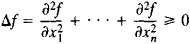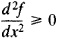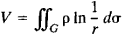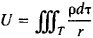# Subharmonic and Superharmonic Functions

## Subharmonic and Superharmonic Functions

A subharmonic function is a function that satisfies in some domain the inequalityWhen Δf = 0, f is a harmonic function. The concept of a sub-harmonic function can thus be regarded as an extension of the concept of a harmonic function.

When n = 1, the condition Δ f ≥ 0 assumes the formIn other words, a subharmonic function of one variable is a convex function. For this reason, the concept of a subharmonic function can be treated as an extension of the concept of a convex function to the case of an arbitrary number of variables. For example, just as any arc of the curve of a convex function lies below the chord joining the end points of the arc, any portion of the surface z = f(x, y) bounded by some closed curve lies below the surface z = F(x, y) passing through that curve if f(x, y) is a subharmonic function of two variables and F(x, y) is a harmonic function; hence the term “subharmonic.”

The above definition assumes that f has second-order partial derivatives. This requirement can be avoided by using the property of the graph of a subharmonic function of lying below the graph of the harmonic function.

A superharmonic function satisfies the inequality Δf≤ 0. If f is a superharmonic function, then –f is a subharmonic function, and vice versa.

The classic examples of subharmonic and superharmonic functions are for n = 2 the logarithmic potentialand for n = 3 the potential function for a volume distribution of charge or masswhere ρ is the mass or charge density. Within the regions G and T these functions satisfy the Poisson equations ΔV = –2πρ and ΔU = –4πρ, respectively, and consequently are superharmonic when ρ ≥ 0 and subharmonic when ρ < 0.

Subharmonic functions are used in solving problems in mathematical physics (in particular, in potential theory) and in the theory of stochastic processes.

### REFERENCE

Privalov, I. I. Subgarmonicheskie funktsii. Moscow-Leningrad, 1937.
Site: Follow: Share:
Open / Close